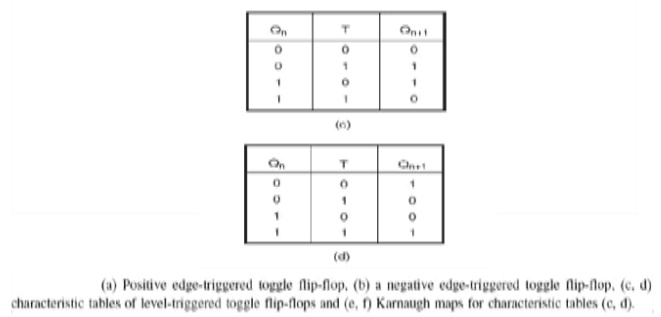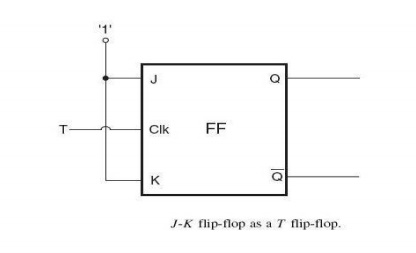Home | | Digital Logic Circuits | JK Flip Flop

# JK Flip Flop

A J-K flip-flop behaves in the same fashion as an R-S flip-flop except for one of the entries in the function table.

J-K Flip-Flop

A J-K flip-flop behaves in the same fashion as an R-S flip-flop except for one of the entries in the function table. In the case of an R-S flip-flop, the input combination S = R = 1 (in the case of a flip-flop with active HIGH inputs) and the input combination S = R = 0 (in the case of a flip-flop with active LOW inputs) are prohibited. In the case of a J-K flip-flop with active HIGH inputs, the output of the flip-flop toggles, that is, it goes to the other state, for J = K = 1 . The output toggles for J = K = 0 in the case of the flip-flop having active LOW inputs. Thus, a J-K flip-flop overcomes the problem of a forbidden input combination of the R-S flip-flop. Figures below respectively show the circuit symbol of level-triggered J-K flip-flops with active HIGH and active LOW inputs, along with their function tables.

The characteristic tables for a J-K flip-flop with active HIGH J and K inputs and a J-K flip-flop with active LOW J and K inputs are respectively shown in Figs 10.28(a) and (b)_ The corresponding Karnaugh maps are shown in Fig below for the characteristics table of Fig and in below for the characteristic table below. The characteristic equations for the Karnaugh maps of below figure is shown nextFIG a. JK flip flop with active high inputs, b. JK flip flop with active low inputs

Toggle Flip-Flop (T Flip-Flop)

The output of a toggle flip-flop, also called a T flip-flop, changes state every time it is triggered at its T input, called the toggle input. That is, the output becomes ‘1‘ if it was 0‘ and ‘0‘ if it was ‘1‘.

Positive edge-triggered and negative edge-triggered T flip-flops, along with their function tables.

If we consider the T input as active when HIGH, the characteristic table of such a flip-flop is shown in Fig. If the T input were active when LOW, then the characteristic table would be as shown in Fig. The Karnaugh maps for the characteristic tables of Figs shown respectively. The characteristic equations as written from the Karnaugh maps are as follows:## J-K Flip-Flop as a Toggle Flip-Flop

If we recall the function table of a J-K flip-flop, we will see that, when both J and K inputs of the flip-flop are tied to their active level (‘1‘ level if J and K are active when HIGH, and 0 level when J and K are active when LOW), the flip-flop behaves like a toggle flip-flop, with its clock input serving as the T input. In fact, the J-K flip-flop can be used to construct any other flip-flop. That is why it is also sometimes referred to as a universal flip-flop. Figure shows the use of a J-K flip-flop as a T flip-flop.Study Material, Lecturing Notes, Assignment, Reference, Wiki description explanation, brief detail
Digital Logic Circuits : Synchronous Sequential Circuits : JK Flip Flop |